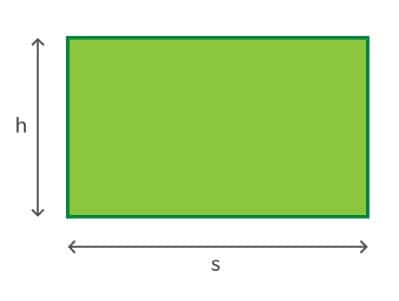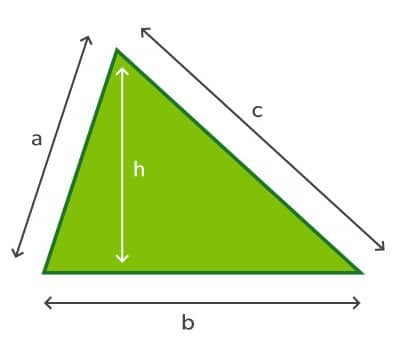# Measuring Turf

## Not sure how much turf you need?

Laying turf doesn’t have to be hard – you just have to get the preparation right and your lawn will be looking amazing in no time. Our simple steps and online turf area calculator makes it easy! It is important that you take care to be accurate.

## How to calculate how much turf you need

1. Get a piece of paper and sketch out the areas to be turfed.
2. Break up the areas into basic shapes such as squares, rectangles and circles. See our full list below.
3. Measure each area and write down your measurements.
4. Calculate how many square metres are in each area and add the totals together. You can use our online turf area calculator below, or see further down how to calculate these manually using some easy formula.
5. Order extra! For simple areas like a rectangle, order 5% extra. For more complex irregular areas, order 10% extra. This will allow for any slight miscalculations and also the odd roll or slab in poor condition that you sometimes get. It’s better to have some turf left over than run out.
6. Calculate the volume of turf underlay that you need; there is some helpful information on how to do this further down this page.

## Online turf area calculator

Once you have measured each part of your lawn, our online turf area calculator will calculate the areas for you and total them together.

## Calculating the area of different shaped areas manually

Once you have measured each part of your lawn, you can calculate the area of each shape manually using these easy formula, not much beyond the simple arithmetic and geometry you probably learned at primary school. Although most of the necessary calculations are generally straightforward, it is nevertheless important that you take care to be accurate. Read on to see how to calculate even the most difficult of shapes.

### SquareMeasure one side of the square, then multiply it by itself (area = s x s)

Example – If the width of the square is 4 metres: 4m x 4m = 16m2

### Rectangle or oblongMeasure the height and width of the square, then multiply them together (area = w x h)

Example – If the height is 4 metres and the width is 6 metres: 4m x 6m = 24m2

### TriangleMeasure the height and base, then multiply the height by half of the base (area = ½b x h)

Example – If the height is 9 metres and the base is 10 metres: 9m x 5m = 45m2

### ParallelogramMeasure the height and base, then multiply together (area = b x h)

Example – If the height is 5 metres and the base is 8 metres: 5m x 8m = 40m2

### TrapezoidMeasure the height and the top and bottom edges. Add the top and bottom edges together (b1 + b2). Divide this figure by 2. Multiple by the height. (area = ½ (b1 + b2) x h)

Example – If the top edge (b1) is 5 metres and the bottom edge (b2) is 9 metres, and height is 6 metres: 5m + 9m = 14m. 14 ÷ 2 = 7.   7 x 6 = 42m2

### Circled = diameter, r = radius Measure the diameter of the circle, then divide this by two to calculate the radius. Multiply the radius by itself, then multiply the total by 3.14. (area = r x r x 3.14) Example – If the diameter is 12 metres, the radius will be 6 metres; multiply 6 metres by 6 metres, then multiply the total by 3.14: 6 x 6 = 36. 36 x 3.14 = 113.04m2

### Ellipse or ovalMeasure the width and height of the oval, then divide each measurement by two to calculate rand r(area =rx r2 x 3.14). Example – If ris 3 metres and ris 5 metres: 3m x 5m = 15m. 15m x 3.14 = 47.1m2

## Calculating the volume of turf underlay

Materials such as soil and sand are measured and ordered in cubic metres and tonnes. To calculate the volume of material required, first work out the area in square metres, and then multiply by the depth to get the cubic metres. Typically you might need 100mm or 150mm of turf underlay (0.1 or 0.15 metres).

Example 1 – You have a rectangle measuring 10m x 6m and need to add 100mm of turf underlay soil. 10m x 6m x 0.1m = 6m3.

Example 2 – You have a circular area with a diameter of 14 metres and a radius of 7 metres, needing 150mm of soil. The area is 7m x 7m x 3.14 = 153.86m2. The volume is 153.86mx 0.15m = 23.079m3, rounded down to 23m3.

Source: https://lawnsolutionsaustralia.com.au/lawn-care/how-to-lay-turf-part-1-measuring/